# 协方差与Matlab的实现

(参考文章)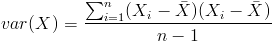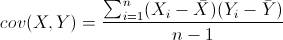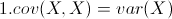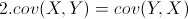Matlab协方差实战

1MySample = fix(rand(10,3)*50)

dim1 = MySample(:,1);dim2 = MySample(:,2);dim3 = MySample(:,3);

sum( (dim1-mean(dim1)) .* (dim2-mean(dim2)) ) / ( size(MySample,1)-1 ) % 得到  74.5333

sum( (dim1-mean(dim1)) .* (dim3-mean(dim3)) ) / ( size(MySample,1)-1 ) % 得到  -10.0889

sum( (dim2-mean(dim2)) .* (dim3-mean(dim3)) ) / ( size(MySample,1)-1 ) % 得到  -106.4000

std(dim1)^2 % 得到   108.3222 std()是求标准差的函数

std(dim2)^2 % 得到   260.6222

std(dim3)^2 % 得到   94.1778

cov(MySample)

=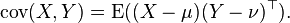X’=[1 2 3 ] %均值是2

Y’=[4 5 6] %均值是5

a=fix(rand(10,3)*50)

a =

37 42 17

12 12 41

25 40 29

34 12 27

44 46 45

47 17 14

27 9 37

6 12 37

7 30 19

12 23 28

x=a-repmat(mean(a),10,1)%这里mean(a)得到的是列平均值，repmat(mean(a),10,1)是产生一个10行一列的矩阵。在这里的一列是指mean(a)整体

x =

11.9000 17.7000 -12.4000

-13.1000 -12.3000 11.6000

-0.1000 15.7000 -0.4000

8.9000 -12.3000 -2.4000

18.9000 21.7000 15.6000

21.9000 -7.3000 -15.4000

1.9000 -15.3000 7.6000

-19.1000 -12.3000 7.6000

-18.1000 5.7000 -10.4000

-13.1000 -1.3000 -1.4000

(x’*x)./ (size(x,1)-1) =

232.9889 70.0778 -31.9333

70.0778 200.6778 -17.2444

-31.9333 -17.2444 111.1556

### 评论 2

1. #0

呵呵 抱歉，现在才回复。谢谢指点，我其实是新手，我稍后会验证下你的说法

huangyanbing02219年前 (2013-07-19)回复
2. #0

不用repmat，用bsxfun函数。在矩阵较大时更节约内存~

Alpha9年前 (2013-06-05)回复
• QQ咨询
• 回顶